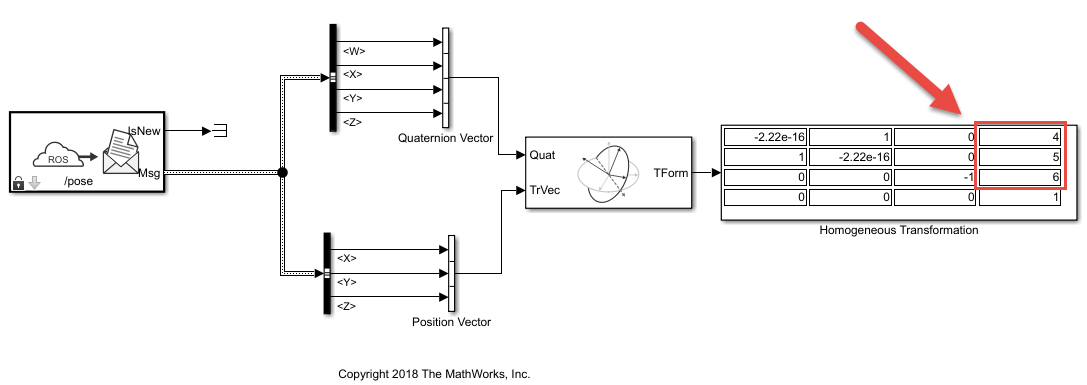# Convert a ROS `Pose` Message to a Homogeneous Transformation

This model subscribes to a `Pose` message on the ROS network and converts it to a homogeneous transformation. Use bus selectors to extract the rotation and translation vectors. The Coordinate Transformation Conversion block takes the rotation vector (euler angles) and translation vector in and gives the homogeneous transformation for the message.

Connect to a ROS network. Create a publisher for the `'/pose'` topic using a `'geometry_msgs/Pose'` message type.

`rosinit`
```Launching ROS Core... Done in 0.91714 seconds. Initializing ROS master on http://192.168.0.10:56207. Initializing global node /matlab_global_node_84807 with NodeURI http://dcc864407glnxa64:39141/ ```
`[pub,msg] = rospublisher('/pose','geometry_msgs/Pose');`

Specify the detailed pose information. The message contains a translation (`Position`) and quaternion (`Orientation`) to express the pose. Send the message via the publisher.

```msg.Position.X = 1; msg.Position.Y = 2; msg.Position.Z = 3; msg.Orientation.X = sqrt(2)/2; msg.Orientation.Y = sqrt(2)/2; msg.Orientation.Z = 0; msg.Orientation.W = 0; send(pub,msg)```

Open the `'pose_to_transformation_model'` model. This model subscribes to the `'/pose'` topic in ROS. The bus selectors extract the quaternion and position vectors from the ROS message. The Coordinate Transformation Conversion block then converts the position (translation) and quaternion to a homogeneous transformation.

For more details, inspect the bus selector in the model to see how the message information is extracted.

`open_system('pose_to_transformation_model.slx')`

Run the model to display the homogeneous transformation.Modify the position or orientation components of the message. Resend the message and run model to see the change in the homogeneous transformation.

```msg.Position.X = 4; msg.Position.Y = 5; msg.Position.Z = 6; send(pub,msg)```

Shutdown the ROS network.

`rosshutdown`
```Shutting down global node /matlab_global_node_84807 with NodeURI http://dcc864407glnxa64:39141/ Shutting down ROS master on http://192.168.0.10:56207. ```